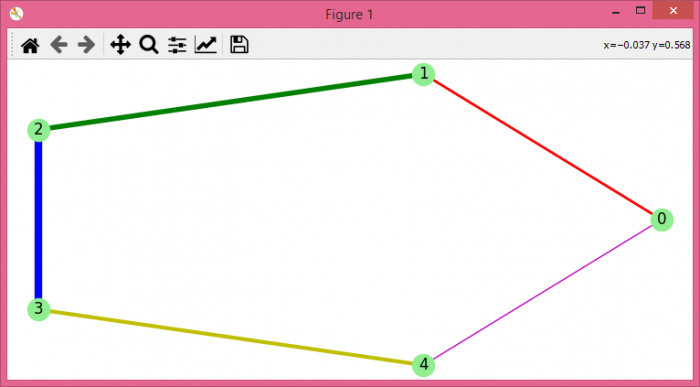# How to change the attributes of a networkx / matplotlib graph drawing?

To change the attributes of a netwrokx/matplotlib graph drawing, we can take the following steps −

## Steps

• Set the figure size and adjust the padding between and around the subplots.

• Initialize a graph with edges, name, or graph attributes.

• Add the graph's attributes. Add an edge between u and v.

• Get the edge attributes from the graph.

• Position the nodes with circles.

• Draw the graph G with Matplotlib.

• To display the figure, use show() method.

## Example

import matplotlib.pyplot as plt
import networkx as nx

plt.rcParams["figure.figsize"] = [7.50, 3.50]
plt.rcParams["figure.autolayout"] = True

G = nx.Graph()

colors = nx.get_edge_attributes(G, 'color').values()
weights = nx.get_edge_attributes(G, 'weight').values()
pos = nx.circular_layout(G)

nx.draw(G, pos,
edge_color=colors,
width=list(weights),
with_labels=True,
node_color='lightgreen')

plt.show()

## Output

It will produce the following output −